Example Questions

← Previous 1

Example Question #61 : Percentage

Harry and Gerry each own equal shares in a gardening company. Their partner, Izzy, owns 40% of the company. What is the percentage increase of the sum of Harry's and Gerry's shares above Izzy's share in the company?

100%

150%

33.3%

50%

20%

50%

Explanation:

This basic percentage problem asks you to determine by what percentage the sum of Harry's and Gerry's share of the company is greater than ("exceeds") Izzy's share. Harry's and Gerry's share's are equal, and the sum is 60% of the company (100% – Izzy's 40% = 60%). 60% is 50% greater than 40% (0.40 * 1.5 = 0.60). Examinees may be tempted to go too far with the problem and mistake their task by finding how much greater Izzy's share is than either Harry's or Gerry's. Be careful to read instructions and translate keywords appropriately.

You may think the answer is 20% (60% – 40%), but this is the difference in terms of total shares in the company, not in terms of Izzy's shares, which is what the question is asking.

Example Question #1 : Percent Of Change

What is the percent change in the area of a circle if its circumference increases from 20 to 45?

225%
406.25%
506.25%
125%
Explanation:

The circumference of a circle increases linearly with its radius.  Therefore, an increase in circumference from 20 to 45 represents an increase of (45 – 20) / 20 = 25 / 20 = 125% increase.  This means that if the radius was r, it is now r + 1.25r = 2.25r.

Now, the original area, supposing r as our radius, would be πr2

The new area would be π(2.25r)2 = 5.0625πr2

The percent change would be: (5.0625πr2 - πr2) / πr2  = (4.0625πr2) / πr2 = 4.0625 or 406.25%

Example Question #1 : How To Find The Percent Of Increase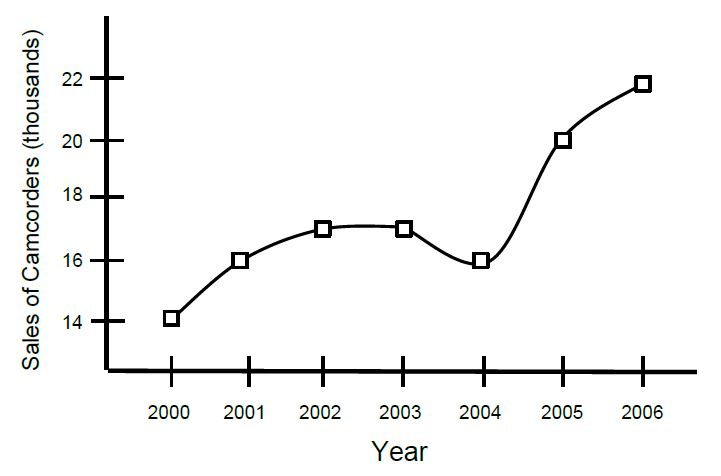According to the graph above, what was the percent change in the sales of camcorders from 2002-2006?

36%

17%

29%

40%

25%

29%

Explanation:

For this problem, pay attention only to the values between 2002 and 2006 - each of which is 17 (thousand) and 22 (thousand). Note that the decrease within this range does not need to be accounted for, as the question asks only for values relative to 2002 and 2006.

To calculate percent change, take it as a fraction: 22/17 = 1.294; this represents approximately 129% of the actual value or a 29% increase in sales across these 4 years.

Example Question #4 : How To Find The Percent Of Increase

What number is 150% greater than 6?

90

3

18

15

9

15

Explanation:

In the problem, 150% of 6 represents the change in the value, not the new value. Percent "greater than" is a key phrase that refers to percent change. Therefore, 9, or 150% of 6, is how much 6 increases. The answer is 6 + 9 = 15.

Formula: Original * (1 + percent increase/100) = New

6(1 + 1.50) = 15

Alex had an investment worth $1500. If the total investment increased 25% the first year and decreased 10% the next year, what is the final worth of the investment. Possible Answers: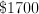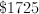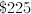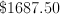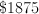Correct answer:Explanation: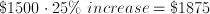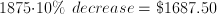Example Question #6 : How To Find The Percent Of Increase Book sales increased by 50% from April 1st to 30th and then decreased by 50% from May 1st to May 31st. Determine the greater quanity. Possible Answers: The quantities are equal The relationship cannot be determined Book sales on April 1st Book sales on May 31st Correct answer: Book sales on April 1st Explanation: If book sales were 100/day then an increase by 50% means they would reach 150/day (by the end of April). A decrease at that point means a 50% decrease in 150/day which would lower book sales to 75/day. Thus sales were higher at the beginning of April than at the end of May. Example Question #7 : How To Find The Percent Of Increase A college student takes out a loan of$8,000 per Quarter. A college student's average living cost is represented by the chart below.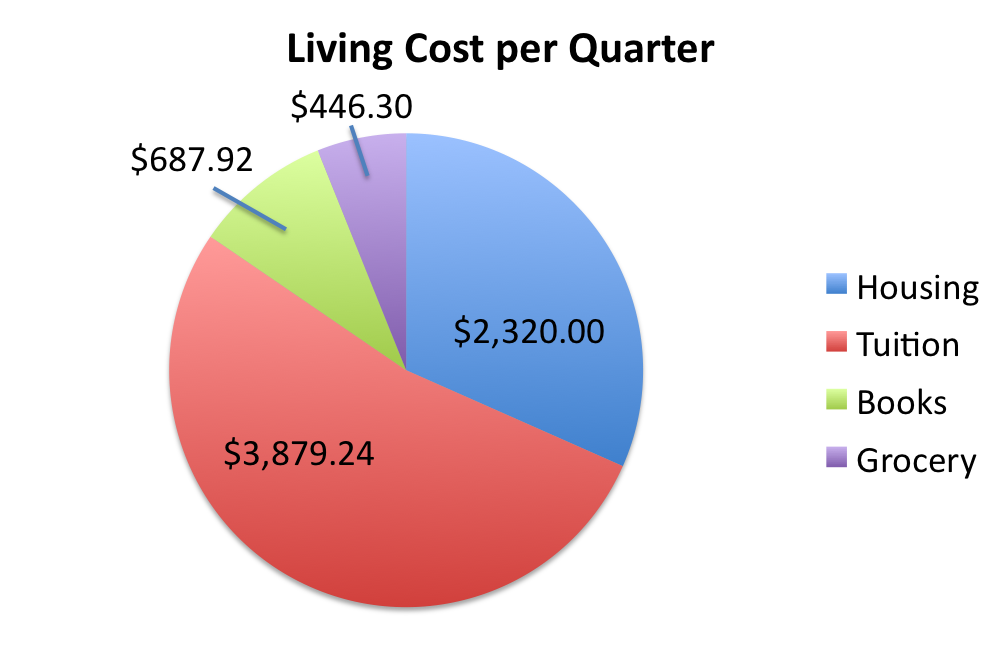Suppose tution is increased by 8%. What is the increase in the percentage of the loan that is spent on tutition and books?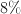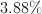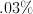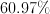Explanation:

The original percentage of the loan spent on tutition and books is 57.09%. After the 8% increase in tutition, the percentage of the loan is 60.97%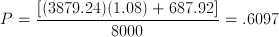Thus, the increase in percentage is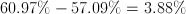.

Example Question #1 : How To Find The Percent Of Increase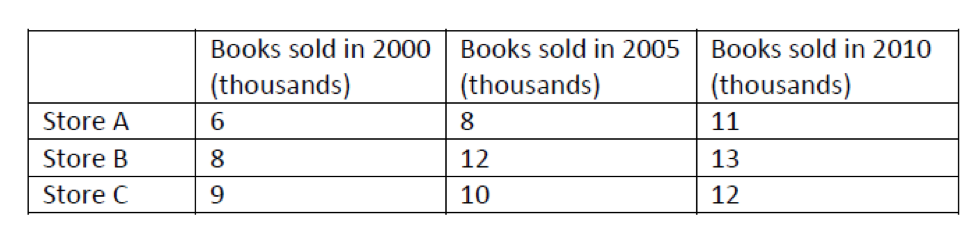By what percentage did the total book sales of the three stores increase from 2005 to 2010?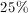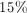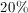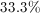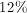Explanation:

Find the total number of books sold in 2005: 8 + 12 + 10 = 30 thousand

Find the total number of books sold in 2010: 11 + 13 + 12 = 36 thousand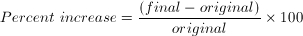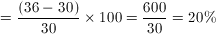Example Question #9 : How To Find The Percent Of Increase

Which of the following is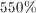greater than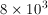?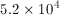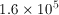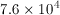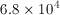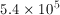Explanation:

The value of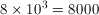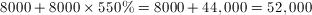.

In scientific notation, this is.

Example Question #10 : How To Find The Percent Of Increase

A new painting company, Paintz, reported a profit of six thousand dollars in 2011. In 2012, Paintz reported \$10,000 more profit than 2011. What was the percentage increase in profit for Paintz between 2011 and 2012?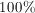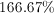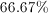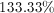%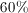Explanation:

The formula for percentage increase is the difference in profit divided by the original profit multiplied by 100. Here the difference in profit between 2011 and 2012 is 10,000 and the original profit in 2012 is 6000, therefore the percentage increase is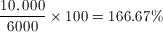.

← Previous 1

Tired of practice problems?

Try live online GRE prep today.